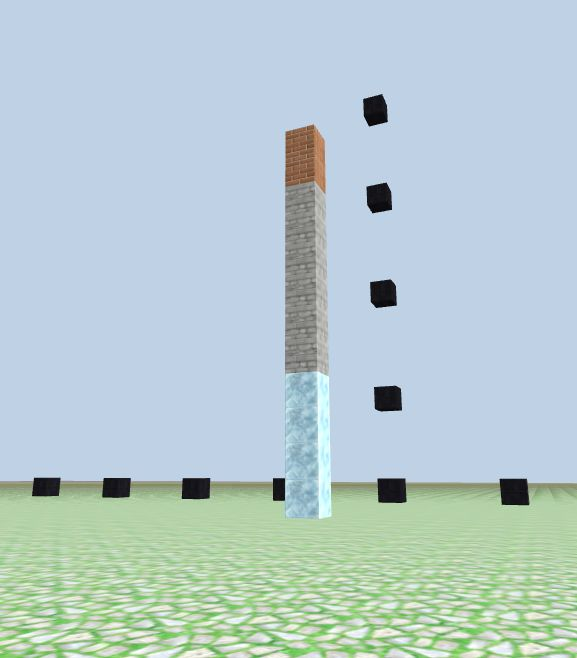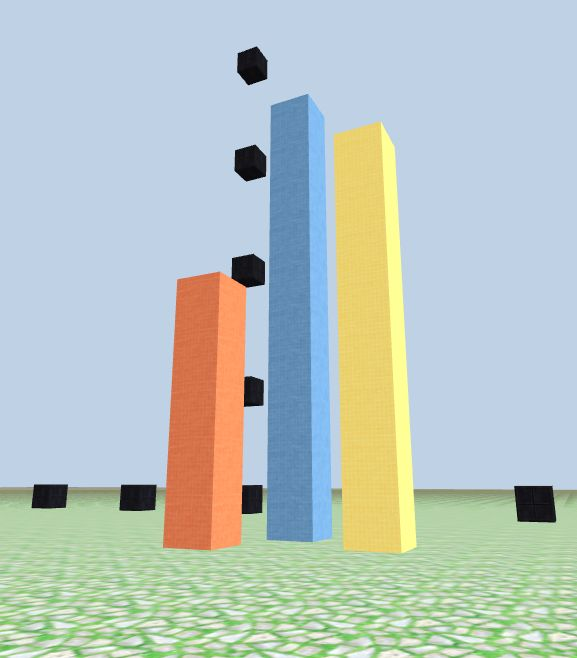### Build columns with function

Build a column Recall that we built a column with for loop?

Let's try make a function out of it:

column10()

A brick column at ground location `x:0, z:-10`, height 10 blocks tall:

``````function column10() {
for (let j = 1; j < 11; j++) {
game.setBlock(new Position(0, j, -10), 17);
}
}

// a 10 blocks tall brick column at (x=0,z=-10)
column10()
``````

Flexible height column: columnH(h)

Add `h` as input argument indicating the column's height:

``````function columnH(h) {
for (let j = 1; j < h+1; j++) {
game.setBlock(new Position(0, j, -10), 17);
}
}

// build some brick columns at (x=0, z=-10) with varying height
columnH(3);
columnH(5);
columnH(10);
``````

Run the above function calls one by one, if you refresh the screen before each `run`, you will see different height columns each time; if you don't refresh, you'll see a column of 3 blocks first, then a 5 blocks tall column replaces the previous shorter one, and a 10 blocks tall column replaces the second one, the column looks getting taller because the taller column covers previous shorter column, these columns are at the same ground location and of the same material.

columnHM(h, m) h: height, m: material

``````function columnHM(h,m) {
for (let j = 1; j < h+1; j++) {
game.setBlock(new Position(0, j, -10), m);
}
}

// some columns with varying height and material
column(12, 17);
column(10, 18);
column(4, 19);
``````

If you don't erase previous structures while running the three functions, what do you expect to see? A column at `x:0, z:-10`, with 3 different colors!columnFlex(x,z,h,m)

Is it time for us to make a `column` function with the most flexibility?

``````// columnFlex(x,z,h,m), h-height, m-material
function columnFlex(x,z,h,m) {
for (let j=1; j <= h; j++) {
game.setBlock(new Position(x, j, z), m);
}
}

// some columns
columnFlex(0,-10,5, 18)
columnFlex(3,-15, 10, 28)
columnFlex(-1,-12, 8, 8)
``````

### Function can call other functions

Function `columnFlex(x,z,h,m)` is good enough in the sense of function flexibility. While in the sense of programming design, reusing code is the spirit. Let's call the previous user-defined function `block_m(x,y,z,m)` to build `column_m(x,z,h,m)`.

Function: column_m(x,z,h,m)

Note: the first two parameters of `column_m` is the ground location `x,z`, then followed by `h,m` (height and material).

``````// block_m(x,y,z, m)
function block_m(x,y,z,m) {
game.setBlock(new Position(x,y,z), m);
}

// column_m(x,z,h,m)
function column_m(x,z,h,m) {
for (let j=1; j <= h; j++) {
block_m(x,j,z,m);
}
}

// some columns
column_m(5,-10, 5, 53);      // orange
column_m(6,-12, 10, 50);     // light blue
column_m(8,-11, 8, 59);     // yellow
``````

Enjoy!